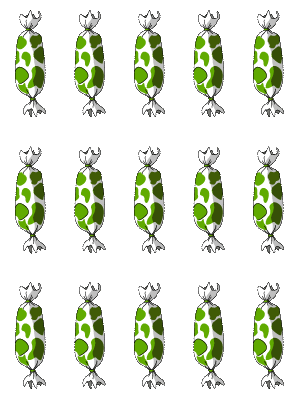# Write Multiplication Expressions for Arrays - Using Arrays To Explain Multiplication Concepts

## Arrays To Show Multiplication Concepts

As we have previously seen, a multiplication sentence consists of three parts; multiplicand, multiplier and the product. In order to write multiplication expressions for arrays, we have to make sure that the array is made up of objects arranged in equal rows and equal columns.

This makes it possible to count the number of columns and to easily multiply the resulting value by the number of rows.
Using arrays to explain multiplication concepts is the best approach in moulding kids for more advanced math.

Moreover, we use arrays to show multiplication concepts because they are the most effective and well-shaped models.

## Click the sections below to find more explanation on this topic

...

### Importance of arrays to multiplication expression

Arrays are the most suitable models that can be used to have a fast and easy understanding of the multiplication expression.

This worksheet has special designed models that enables kids to have an interest in knowing the number of object both on the vertical and horizontal lines.

Thus a sweet and convenient knowledge to write multiplication expressions for arrays.
In addition to this, when a product or a factor in a multiplication sentence is unknown, it becomes very easy for kids to use arrays to obtain an answer.

### Example: use arrays to complete multiplication sentences

Consider an array of 3 rows and 5 columns of sweets.Complete the multiplication sentence below that describes the array.

___ x 5 = 15

It is very easy.

From our knowledge of the parts of a multiplication sentence, we've noticed that the multiplicand (5) and the product (15) are available in the multiplication sentence above, while the multiplier is unknown.

### How then can the multiplier be obtained using arrays?

• We begin by first of all counting the number of sweets in our first column. 'Yes! There are 5 sweets'.
Ooops! 5 is the multiplicand.
• Secondly, we count the number of sweets on the first row.
'Yes! I've got 3 sweets'.
'Ah! There's no 3 in the multiplication sentence'.
Therefore, '3 is the multiplier'. So, 'I'll fill my box with 3.

5 x 3 = 15

Huraaay! I love to use arrays again.

I got my answer so easily.